﻿ A Multivariate Time Series Modeling of Major Economic Indicators in Nigeria

### A Multivariate Time Series Modeling of Major Economic Indicators in Nigeria

C. E Onwukwe, G. O. NwaforOPEN ACCESSPEER-REVIEWED

## A Multivariate Time Series Modeling of Major Economic Indicators in Nigeria

C. E Onwukwe1,, G. O. Nwafor2

1Department of Mathematics, Statistics and Computer Science University of Calabar, P.M.B 1115, Cross River State, Nigeria

2Department of Mathematics and Statistics Cross River University of Technology Calabar, Nigeria

### Abstract

One of the key objectives of every good economy, whether or not developing or developed is to achieve a high and sustainable economic growth rate coupled with the economic indicators. The research on the Multivariate Time Series Modeling of Major Economic Indicators in Nigeria, aims at providing quantitative analysis of the dynamics on currency in circulation, exchange rate, external reserve, gross domestic product, money supply and price deflator. This study utilizes secondary data obtained from the Central Bank of Nigeria, Statistical Bulletin (vol. 21: 2010), of all variables investigated in the model. The sample covers quarterly data from 1981 to 2010. The study employed the newly developed multivariate time series estimation technique via Vector Autoregressive modeling to model the economic indicators in Nigeria. The empirical result yields a stable and sustainable economic model for the six economic variables in the study. The Granger causality analysis indicates that there exists unidirectional and bidirectional causality between the economic variables. Gross domestic product and external reserve was seen as a good predictor to other economic indicators. The relationship between these economic indicators is however significantly determined which is positive in either direction. The empirical model provides forecast value for the next two years.

### At a glance: Figures

1
Prev Next

• Onwukwe, C. E, and G. O. Nwafor. "A Multivariate Time Series Modeling of Major Economic Indicators in Nigeria." American Journal of Applied Mathematics and Statistics 2.6 (2014): 376-385.
• Onwukwe, C. E. , & Nwafor, G. O. (2014). A Multivariate Time Series Modeling of Major Economic Indicators in Nigeria. American Journal of Applied Mathematics and Statistics, 2(6), 376-385.
• Onwukwe, C. E, and G. O. Nwafor. "A Multivariate Time Series Modeling of Major Economic Indicators in Nigeria." American Journal of Applied Mathematics and Statistics 2, no. 6 (2014): 376-385.

 Import into BibTeX Import into EndNote Import into RefMan Import into RefWorks

### 1. Introduction

Statisticians and Econometricians do four things: describe and summarize data, make forecasts, quantify what the researcher did and did not know about the time structure of the economy, and advise (and sometimes become) policy makers. In the 1970s, these four tasks – data description, forecasting, structural inference, and policy analysis – were performed using a variety of techniques. These ranged from large models with hundreds of equations, to single equation models involving only a single variable. Like many countries, industrialized and developing one of the most fundamental objective of macro-economic policies in Nigeria is to sustain high economic growth with good measure of major economic indicators. There exists a considerable debate regarding the existence and Gross domestic product (GDP) and other economic indicators. A consensus nevertheless exists thus suggesting that macro-economic stability, which is rooted in the spirit of good measure of GDP, is positively related to other economic indicators.  Implicitly recognizes exchange rate, money supply and Gross Domestic Product (GDP) as a major economic indicator over time. In recent times, the Central Bank of Nigeria (CBN) bulletin  reviews data on the external reserve, price deflator and currency in circulation etc. Multivariate time series (MTS) data are widely available in different fields including medicine, finance, science and engineering. Modeling MTS data effectively is important for many decision making activities. Consider a time series variable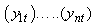. A multivariate time series is the (n x 1) vector time series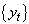where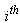row of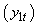is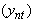, that is for any time t.

Multivariate time series analysis is used when one wants to model and explain the interactions and co-movements among a group of time series variables (Economic indicators). Multivariate methods are very important in economics and much less so in other applications of forecasting. The multivariate view is central in economics, where single variables are traditionally viewed in the context of relationship to other variables.

In forecasting and even in economics, multivariate models are convenient in modeling interesting interdependencies and achieve a better fit within a given data or economic indicator. Multivariate forecasting methods rely on models in the statistical sense of the word, though there have been some attempts at generalizing extrapolation methods to the multivariate case. This does not necessarily imply that these methods rely on models with regards to whether they are a theoretical, such as time series models, or structural or theory-based. Much research has gone into the development of ways of analysis multivariate time series (MTS) data in both the statistical and artificial intelligence communities. Statistical Multivariate Time Series modeling methods include the vector Auto-regressive process.

In this research, the interest is to model the major economic indicators (currency in circulation, exchange rate, external reserve, gross domestic product, money supply and price deflator) in Nigeria with the approach of vector Auto-regressive (VAR) process detailed on endogenous variable (dependent) over time period from (1981 - 2010) in Nigeria.

### 2. Material and Methodology

2.1. Data Collection Technique

The data type and source of this paper mainly, the secondary macroeconomic time series data in its analysis. All data used in the analysis was sourced from Central Bank of Nigeria Statistical Bulletin . Other augmenting sources of this study will include published articles and journals, working papers, textbooks and relevant internet resources.

2.1.1. Unit Root Test

A number of alternative tests are available for testing whether a series is stationary or not, the Augmented Dickey – fuller (ADF),  for (ADF) test, where k is chosen to ensure that the residuals follows a pure random process. ADF unit root, tests the null hypothesis is that the series is not stationary and this is either accepted or rejected by examination of the t-ratio of the lagged term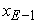compared with the tabulated values. If the t-ratio is less than the critical value the null hypothesis of a unit root (i.e. the series is not stationary) is accepted. If so the first difference of the series is evaluated and if the null hypothesis is rejected the series is considered stationary and the assumption is that the series is integrated of order one 1(1).

The ADF regression test is as follows: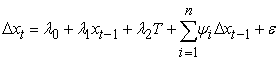(1)

Where Δ is the difference operator.

x is the natural logarithm of the series

T is a trend variable

λ and Ψ are the parameters to be estimated and

ε is the error term

2.1.2. Model Specification

Model specification in the present context involves selection of the VAR order and in VECM also choosing the cointegration rank. Lutkepohl  because the number of parameters in these models increases with the square of the number of variables it is also often desirable to impose zero restrictions on the parameter matrices and thereby eliminates some lagged variables from some of the equations of the systems.

2.1.3. Model Selection Criteria

The standard model selection criteria which are used in this context chosen the VAR order which minimizes them over a set of possible orders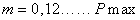. The general form of a set of such criteria is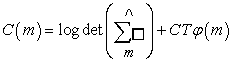Whereis the residual covariance matrix estimator for a model of order m. φ(m) Is a function of the order m which penalizes large VAR orders. CT is a sequence which may depend on the sample size and identifies the specific criterion. The term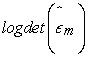is a monincreasing function of the order m which φ(m) increases with m. The lag order is chosen which optimally balances these two forces. , to estimate the system, the order p i.e. the maximal lag of the system has to be determined. The multivariate case with k variables, T observations, a constant term and a maximal lag of p, these criteria are as follows:

Final prediction error (FPE)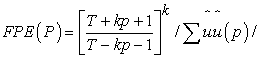Akaike information criterion (AIC)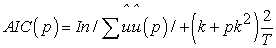Hannan – Quinn criterion (HQ)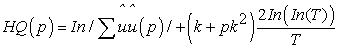Shwarz criterion (SC)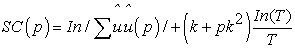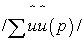is the determinant of the variance covariance matrix of the estimated residuals. , AIC suggests the largest order, SC chooses the smallest order and HQ is in between. The choice of the best model is based on the model selection criteria, the minimum model selection criteria compared to others.  VAR methodology superficially resembles simultaneous equation modeling in that it considers several endogenous variables together. But each endogenous variable is explained by its lagged, or past, values and the lagged values of all other endogenous variables in the model, usually, there are no exogenous variables in the model.

2.1.4. The levels VAR Representation

, “if the is true simultaneity among a set of variable, they should all be treated on an equal footing, there should not be any a priori distinction between endogenous and exogenous variables”. It is in this spirit that Sims developed his VAR model.

The stochastic part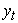is a assumed to be generated by a VAR process of order p (VAR (p)) of the form.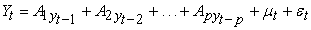(3)

Where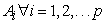are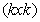parameter matrices.

The error process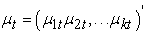is a k – dimensional zero mean white noise process with covariance matrix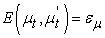.

In matrix notations the m time series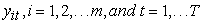Where

t is the common length of the time series.

Then a Vector Autoregressive Model is defined as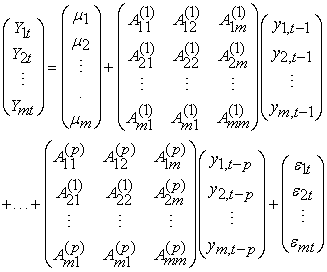Where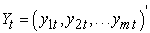Denote (nx 1) vector of time series variables

Ai are (nx n) coefficient matrices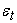Is an (nx 1) unobservable zero mean white noise vector process.

### 3. Estimation Results

3.1. Unit Root Test Results

In establishing that there exist long run relationships amongst the variables, and to avert false result, the order of integration of each of the variable is inspected using the Augmented Dickey-Fuller (ADF) unit roots tests. Before performing these tests, an informal inspection of the trends in the logarithmic levels and differences is done. The plot of each of the series indicates that the variables are non stationary at their levels. However, the first difference indicates that the variables are stationary. Though, the time plot examination provides some insights into the order of integration of the variables, a formal test is appropriate.

Thus, Table 1. presents the results of the unit root test using the ADF tests. The results indicate that, each of the series is integrated of order one (that is each series is I(1)) and thus contain unit root. The results of the unit root test corroborate the graphical test that all the series are non-stationary at their levels. It is relevant to stress that, achieving stationarity is a precondition for the estimating of VAR model and consequently Granger causality analysis.

#### Table 1. Augmented Dickey-Fuller Unit Root Test

3.1.1. VAR MODEL

The Vector Autoregressive Model is presented in matrix form below: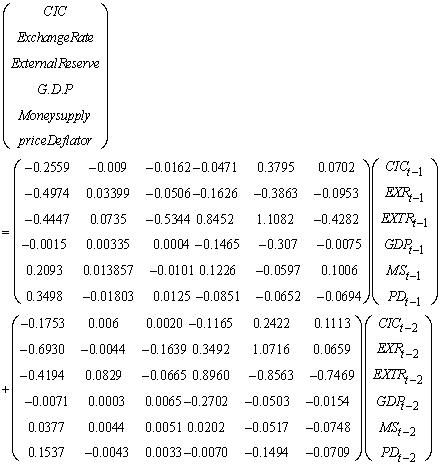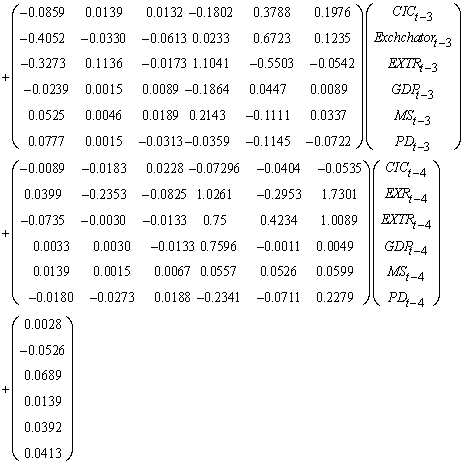By equation we obtained that: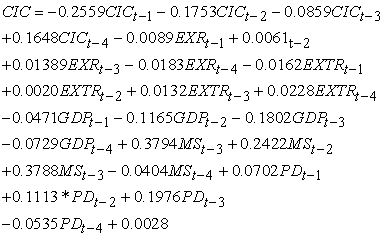(1)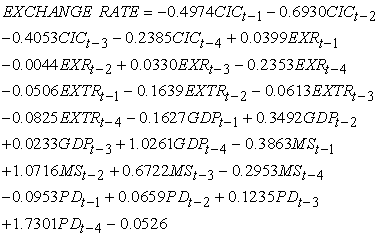(2)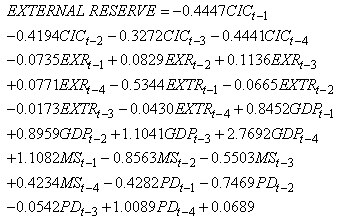(3)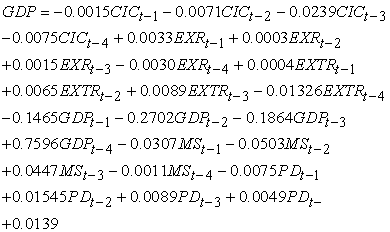(4)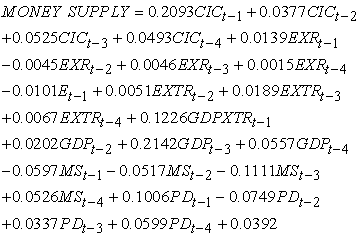(5)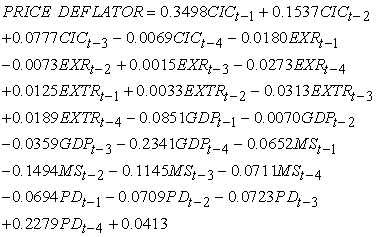(6)

3.1.2. Roots of Characteristics Polynomial

The system is stable at the model 4 of all included variables. The above result indicates that the eigen values of the system obtainable in modulus lies within the unit circle. The roots of characteristics polynomial does not lies outside the unit circle: Hence from the analysis VAR (4) satisfies the stability condition.

Figure 1. AR Characteristic Polynomial of the endogenous graph of the all the economic variable

#### Table 3. Summaries of Pair Wise Granger Causality Tests

The results indicate that exchange rate Granger causes Currency in circulation. Thus past Exchange rates can be used to predict future currency in circulation. However, Gross Domestic product Granger causes currency in circulation and verse versa. The results further show that, GDP Granger causes Exchange rate and can be used to predict future exchange rate activities. Specifically, money supply, external reverse, price deflator and Gross Domestic Product Granger causes each other, therefore there exist bidirectional causality between them.

Currency in circulation, money supply, price deflator and exchange rate has a clear picture of unidirectional causality between them.

There is an obvious indication that Gross Domestic Product and External Reverse are key in determining the activities pertaining to the economy of Nigeria. These variables are sensitive and hence affect every sector of the economy. It is therefore not surprising that exchange rate as to the Nigeria economy is gradually devalued.

### 4. Discussion

Multivariate Time Series (MTS) data are widely available in different field including medicine, finance, science and engineering. , modeling multivariate time series (MTS) data effectively is important for many decision making activities. Macro-economic practitioners frequently work with multivariate time series models such as Vector autoregressive (VAR), Vector error correction model (VECM), and factor augmented Autoregressive (ARs) as well as time-varying parameter.

In this paper, the review of the theoretical and empirical studies that investigate the relationship among exchange rate, money supply, gross domestic product (GDP) and several economic indicators is done. Extensive theoretical and empirical work has been devoted to the study of these variables (economic indicators).  Standard practice in VAR analysis is to report results from Granger – causality test, impulse responses and forecast error variance decompositions. These statistics are computed automatically (or nearly so) by many econometrics and statistics packages (R, E-views and SPSS). Because of the complicated dynamics in the VAR, these statistics are more informative.  examine the link between the naira depreciation, inflation and output in Nigeria, adopting vector autoregressive (VAR) and its exchange rate system does not necessarily lead output expansion, particularly in short term. Issues such as discipline, confidence and credibility on the part of the government are essential. Evidence from impulse response functions and structural VAR models suggested that the impacts of the lending rate and inflation on the output were negative. While most previous studies focus more on the determinants of inflation, using explanatory variable, our deviates by adopting the vector error correction mechanism (VECM) which eliminates the need to develop explicit economic models and thus impose a prior restrictions on the relationships among different economic variables than is possible in conventional econometric analysis.  explores the identified vector autoregressive (VAR) to model the relationship between CPI, money supply and exchange rate in Ukraine. The results show that exchange rate shocks significantly influence price level behavior. Further, the study also found that money supply responds to positive shocks in price level. The study contributes to the sizable literature on IT using overly sophisticated vector error correction model (VECM) with complex identification structure.

4.1. Varieties of VAR

VARs comes in three varieties, 

A Reduced Form VAR: Expresses each variable as a linear function of its own past values, the past values of all other variables being considered, and a serially uncorrelated error term. Thus, in our example, the VAR involves three equations: current unemployment as a function of past values of unemployment, inflation and the interest rate, inflation as a function of past values of inflation, unemployment and the interest rate: and similarly for the interest rate equation. Each equation is estimated by ordinary least squares (OLS) regression.

A Recursive VAR: Constructs the error terms in the each regression equation to be uncorrelated with the error in the preceding equations. This is done by judiciously including some contemporaneous values as regressors. Consider a three-variable VAR, ordered as (i) inflation, (ii) the unemployment rate, (iii) the interest rates. In the first equation of the corresponding recursive VAR, inflation is the dependent variable and the regressors are lagged values of all three variables.

A Structural AR: Uses economic theory to sort out the contemporaneous links between the variables ([12, 13, 14]). Structural VARs require ‘indentifying assumptions’ that allow correlations to be interpreted causally. These indentifying assumptions can involve the entire VAR, so that all of the causal links in the model are spelled out, or just a single equation, so that only a specific causal link is identified.  the estimated effects of a momentary policy shock on the rates of inflation and unemployment depends on the details of the presumed momentary policy rule. In other words, the estimates of the structural impulse hinge on detailed institutional knowledge of how the fed set interest rates. Of course, the observation that results depend on assumption is hardly new. The operative question is whether the assumptions made in VAR models are any more compelling than in other econometric models. This is a matter of heated debate and is thoughtfully discussed by [1, 16] and [17, 18] and . Here are three important criticisms of structural VAR modeling.

(i) What really makes up the VAR “shocks”? In large part, these shocks, like those in conventional regression, reflect factors omitted from the model. If these factors are correlated with the included variables then the VAR estimates will contain omitted variable bias. For example, fed officials might scoff at the idea that they mechanically followed a Taylor rule, or any other fixed – coefficient mechanical rule involving only a few variables, rather, they suggest that their decisions are based on a subtle analysis of very many macroeconomic factors, both quantitative and qualitative. These considerations, when omitted from the VAR, end up in the error term and (incorrectly) become part of the estimated historical “shock” used to estimate an impulse response. A concrete example of this in the VAR literature involves the “price puzzle”. Early VARs showed odd result inflation tended to increase following monetary policy tightening. One explanation for this  was that the fed was forwarding looking when it set interest rates and that simple VARs omitted variables that could be used to predict future inflation, when these omitted variables intimated an increase in inflation, the fed tended to increase interest rates. Thus these VAR interest rate shocks presaged increases in inflation. Because of omitted variables, the VAR mistakenly viewed labeled these increases in interest rates as monetary shocks, which led to biased impulse responses.

(ii) Policy rules change over time, and formal statistical tests reveal widespread instability in low-dimensional VARs . Constant parameter structural VARs that miss this instability are improperly identified. For examples, several researchers have documented instability in monetary policy rules (for example, ),  and , and this suggests misspecification in constant coefficient VAR models (like our three-variable example) that are estimated over long sample periods.

(iii) The timing conventions in VARs do not necessarily reflect real-time data viability, and this undercuts the common method of identifying restrictions based on timing assumptions. For example, a common assumption made in structural VARs is that variables like output and inflation are sticky and do respond “within the period” to monetary policy shocks. This seems plausible over the period of a single day but becomes less plausible over a month or quarter. Until now, we have carefully distinguished between recursive and structural VARs recursive VARs use an arbitrary mechanical method to model contemporaneous correlation in the variables, while structural VARs use economic theory to associate these correlations with causal relationships. Despite these criticisms we think it is possible to have credible identifying assumptions in a VAR. one approach is to exploit detailed institutional knowledge. An example, of this is  study of the macroeconomic effects of fiscal policy (taxes and government spending). They argue that the tax code and spending rules impose tight constraints on the way that taxes and spending vary within the quarter, and they use these constraints to identify exogenous in taxes and spending necessary for causal analysis. A different approach to identification is to use long run restrictions to identify shocks, for example  use the long –run neutrality of money to identify monetary shocks. However, assumptions based on the infinite future raise questions of their own [26, 27], an exchange rate is the price of one currency, given in terms of another. The movement of a currency’s value relative to others has a profound effect on economies exposed to this currency. Given the interlinked nature of modern economies, exchange rate movements have the power to profoundly affect business, governments, and people around the globe. The spot rate is also referred to as the normal exchange rate. The nominal exchange rate measures the value of one currency in terms of another and it the indirect quotation. , several factors could cause exchange rate changes. These include change in foreign exchange supply and demand, balance of payments problems, rising inflation, interest rate national income, monetary supervision, changing expectations and speculation. , used two partial models to investigate the impact of investment on GDP growth rate and the relationship between interest rate and investment in the case of the Romanian economy. , estimated the relationship between the annual rate of economic growth (YC) and the real rate of interest (RR) in equations of the basic form.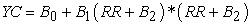(4)

The study shows the effect of a rising real interest rate on growth and claimed that growth is maximized when the real rate of interest lies within the normal range -5% to +15%. , examines monetary policy in Albania during the transition periods. Estimates from a vector autoregressive model (VAR) of key macroeconomic variables which include money growth, inflation, exchange rate, remittance and the trade balance, demonstrate the weak link between money supply and inflation up to mid-2000.  Extend evidence on the lag between monetary policy actions and the response of inflation. Their evidence is based on UK and US data for the period 1953 – 2001 on money growth rate, inflation and interest rates, as well as annual data on money growth and inflation.  and  consider relations between both money supply and inflation and between money supply and GDP. Their findings confirm a long – run relationship between money growth and inflation. The period of money expansion and high inflation in decade of 1990’s was accompanied by contraction of output.  also discusses this issues taking note of the break point in the statistical relationship. In a more recent period of financial stability (1999 - 2003) rising monetary aggregate were accompanied by falling inflation and a rebound of output. , note that nominal exchange rate stability could contribute to moderate growth rates of prices during the last few years.  empirically estimate and test the relationship between exchange rate accommodation and the degree of inflation persistence using a non-linear auto-regressive inflation equation for ten European countries for the period 1974:1 – 1998: 2. In the estimation procedure they allow for the presence of an unknown number of shifts in the mean of inflation. Their results provide supportive evidence for the existence of a positive link between exchange rate accommodation and inflation persistence for most of the smaller and more dependent exchange rate mechanism (ERM) countries, even when mean level shifts in inflation are appropriately accounted for.  discusses whether inflation forecasts should be based on technical exchange rate and uncovered interest rate parity (ULP) or no assumption reflecting the central bank’s best prediction of future exchange rate movements. Because of the strong link between the interest rate and the e exchange rate, the exchange rate does not principally differ from other variables that are endogenous in inflation projections. , argues that along with change in output growth, exchange rate changes have historically played a key role in the adjustment of external imbalances industrial countries. [39, 40] finds that, a surprise monetary policy shock that increase the interest rate has a significant appreciating effect on the exchange rate.  Advantage of providing an observable commitment to monetary policy. They formalize the argument that because it is more transparent, the exchange rate has a natural advantage as an instrument for monetary policy. , explore the relationship between monetary growth, exchange rate and inflation in Ghana using error correction mechanism (ECM). The empirical result confirms the existence of a long run equilibrium relationship between inflation, money supply, exchange rate and real income. In line with theory, the findings demonstrate that in the long-run, inflation in Ghana is positively related to the money supply and the exchange rate, while it is negatively related to real income.  Separated the influence on monetary growth from exchange rate changes on prevailing and predicted rates of inflation. The sample covers ten African countries: The Gambia, Ghana, Kenya, Nigeria, Sierra-Leone, Somalia, etc. using the vector auto-regression analysis, they suggest that monetary dynamics dominate inflation level, in four countries while in three countries, exchange rate depreciations are the dominant factor.

### 5. Conclusion

The study on the Multivariate Time Series Modeling of Major Economic Indicator in Nigeria reveals some facts about the economy of Nigeria for the period under investigation. The research obtained a stable model for the vector autoregressive for the six economic variables. The result of the Granger causality analysis is unique enough, and there exist causality between variables. The Exchange rate as at the period of study reveals weak correlation which signifies the weak and devaluation of the Nigeria currency. Gross Domestic Product was seen as a good predictor to other economic indicator and the External Reverse. The relationship between these economic indicators is however significantly determined which is positive in either direction. This implies that the connection among these economic indicators and economic activities in Nigeria over some period of time is not immediately automatic and the study also provides forecast value for the next two years from the last period of investigation.

5.1. Policy Implication and Recommendations

The policy implication is that the economy of Nigeria is quite responsive to specifically the economic indicators mentioned in this study. Consequently, predicting changes in the economic indicators by economic factors is of great importance in decision making. The fact is that regarding GDP and External Reverse, the predictive ability is actually in order. Policy direction consequent upon the empirical results is to sensitize the Central Bank and Economic policy makers to carefully define the monetary policies which affects some of these key economic indicators in Nigeria particularly Exchange Rate.

### References

  Akinbola, T. O. “The dynamics of money supply, exchange rate and inflation in Nigeria”. Journal of Applied Finance and Banking. Vol. 2 No 4 2012: 117-141. 2012.In article  CBN “Central Bank of Nigeria Statistical bulletin” CBN Nig. Vol. 21, Dec, 2010.In article  Dickey, D. A. and Fuller, W. A. “Distribution of the estimators for Autoregressive time series with a unit root”. Journal of the American Statistical Association. 74: 427-431. 1969.In article  Lutkepohl Helmut “Econometric Analysis with Vector Autoregressive models” European university institute, Florence. 2007.In article  Gebhard K and Jurgen W. “Introduction to modern Time series Analysis” Springer Berlin Heidelberg New York. 2007.In article  Damodar, N. Gujarati “Basic Econometrics, fourth edition”. McGraw-Hill companies, New York. 2004.In article  Christopher, A. Sims “vector Auto regression” Vol. 48, No 1. (Jan, 1980). Pp 1-48 (www.pauldeng.com./.../).Springer Berlin Heidelberg, New York. 1980In article  James, H. S and M, W. Watson. “Vector Auto regression” National Bureau of Economic Research, Cambridge Massachusetts. 2001.In article  Liu, X., Swift, S., Tucker, A., Cheng, G. and Loizou, G. “Modelling multivariate time series”. Birkbeck College, university of London Mallet Street, London WCIE 7Hx, UK (www.ifs.tuwien.ac.at/.../idamap99.09.pdf). 2012.In article  Odusola A. F and Akinlo. A. E “output inflation and exchange rate in developing Countries” An application to Nigeria. 2001.In article  Dmyto, Holod. “The relationship between price level, money supply and exchange rate in Ukraine” A thesis submitted to the National University of keiv-mohyla Academic. 2000.In article  Bernanke, B. S. “Alternative Explorations of the Money-Income Correlation” Carnegie-Rochester Conference Series on Public policy. 25: 49-99. 1986.In article CrossRef  Blanchard, O. J. and Mark, W. W. “Are Busienss Cylces All Alike?” in The American Business Cycle. R. J. Gordon Editor. Chicago: university of Chicago Press. 1986.In article  Sims, C. A “Macroeconomics and Reality”. Econometric. 48: 1-48. 1980.In article CrossRef  Leeper, E. M., Christopher, A. S. and Tao, Z. “What Does Monetary Policy Do?” Brookings Papers on Economic Activity. 1996 (2): 1-63.1996.In article  Christiano, L. J., Martin, E. and Charles, L. E. “Monetary Policy Shocks: What Have What learned and to what End”? in Handbook of Macroeconomics. Vol. 1A Chapter 2, 65-148. 1999.In article  Cochrane, J. H. “What do the VARs Mean? Measuring the Output Effects of Monetary policy”. Journal of Monetary Economics. 41: 2, 277-300. 1998.In article CrossRef  Rudebusch, G. D. “Do Measures of Monetary Policy in a VAR Make Sense”? International Economic Review. 39: 907-931. 1998.In article CrossRef  Sims, C. A. “Interpreting the Macroeconomic Time Series Facts: The Effects of Monetary policy”, European Economic Review: 36: 975-1011. 1992.In article CrossRef  Stock, J. H. and M, W. Watson. “Evidence on Structural Instability in Macroeconomic Time Series Relations”. Journal of Business and Economic Statistics. 14: 11-29. 1996.In article  Bernanke, B. S. and Alan, B. “The Federal Funds Rate and the Channels of Monetary Transmission”. American Economic Review. Vol. 82 no 4 pp 901-21. 1992.In article  Clarida, R., Jordi, G. and Mark, G. “The Science of Monetary Policy”. A new Keynesian perspective” Journal of Economic Literature 37: 1661-1734. 1999.In article CrossRef  Boivin, J. “The Fed’s Conduct of Monetary Policy: Has it Changed and Does in Matter”. Manuscript, Columbia University. 2000.In article  Blancard, O. J. and Perotti, R. “An Empirical Characterization of the Dynamic Effects of Changes in Government Spending and Taxes on Output” NBER Working Paper. 1999.In article  King, Robert G, Charles. I. Plosser, Jame. H. Stock and Mark. W. Watson “stochastic trends and economic fluctuation.” American Economic Review. 81, no. 4 pp. 819-840. 1991.In article  Faust, J. and Eric, M. L. “When Do Long-Run Identifying Restrictions Given Reliable Results”. Journal of Business and Economics Statistics. 345-353. 1997.In article  Ansong, M. R. “A multivariate Time series data analysis on real economic activity data” GHANA. M.Sc dissertation. 2013.In article  Elias, C. and Joshua, G. “Monetary growth and exchange rate depreciation as causes of inflation in African countries” international monetary fund, Washington, DC, world Bank working paper. 2000.In article  Albu, L. “Trends in the interest rate-investment-GDP growth relationship”. Romanian J. Econ. Forecast No. 2. 2006.In article  Oosterbaan, M. S., Der, Windt, N. V. and Steveninck, T. R. V. “Determinants of growth” (ed). Available at http://books.google.co.uk/books. 2000.In article  Marta, M.; Peter, S. and Anita, T. “Inflation, exchange and the role of monetary policy in Albania” working paper. 88. 2004.In article  Nicolleta, B. and Edward, N. “The lag from the monetary policy actions to inflation” fried may revisited external MPL Unit Discussion paper. 2001.In article  Tyrkalo. R and Adamyk. B “monetary policy transmission and its meaning to the Effectiveness of NBU activities” Herald of NBU, 7. 1997.In article  Doroshenko. I “Monetary policy of economic growth in the transitional economy, Shevchenko national policy of economic university Kyir” Ph.D. dissertation. 2001.In article  Oksana, N. and Mykyta, M. “Ukainian money market, mechanism of monetary policy influence on the economy” CASE-Ukraine, Kyir. 2004.In article  Clemens, J. M. K. and Alex, L. “Inflation persistence under semi-fixed exchange rate regimes” The European evidence, 1974-1998. 2002.In article  Bernhandsen, T. and Holnsen, A. “The choice of exchange rate assumption in the process of forecasting inflation” staff memo, 3, central bank of novway. 2005.In article  Debelle, G. and Galati, G. “Current account adjustment and capital flows” Bls working paper. 169. 2005.In article  Zettelmeyer, J. “The impact of monetary policy on the exchange rate” Evidence from three small open economics, Journal of Monetary Economics. 51: 635-652.2004.In article  Kearn, J. and Manners, P. “The impact of monetary policy on the exchange rate” A study Intra-dat Data, Reserve Bank of Australia, Research Discussion paper. 2005.In article  Andrew, A. and Patrick, K. “The advantage of transparent instruments of monetary policy, federal reserve Bank of mineapolis” research department staff report. 297. 2001.In article  Mahamadu, B. and phillip, Abradu-Otoo. “Monetary growth, exchange rates and inflation in Ghana” An error correction analysis, working paper WP/BOG 2003/05. 2003.In article

### APPENDIX 1

Figure 2. Residuals graphs displays a separate graph of the residuals from each equation in the system

### APPENDIX 2

For each model, forecasts start after the last non-missing in the range of the requested estimation period, and end at the last period for which non-missing values of all the predictors are available or at the end date of the requested forecast period.

### APPENDIX 3

#### Table 5. Pair – Wise Correlation of the Variables

The result in Table 5 Pair – Wise Correlation of the Variables indicates that currency in circulation is positively correlated with other key economic indicators. However, the correlation is somewhat weak with regards to exchange rate with 0.2326. Exchange rate has a poor positively correlated with other key indicators in Nigeria. Currency in circulation, external reserves, GDP, money supply and price deflator are positively correlated with the magnitude indicating a high correlation. It is however, important to emphasize that, correlation does not necessarily imply causation. The poor correlation of the exchange rate signifies the devaluation of the Naira as to compare with foreign currencies.